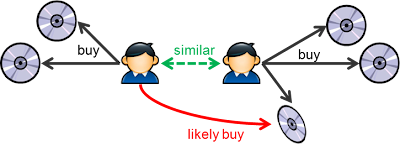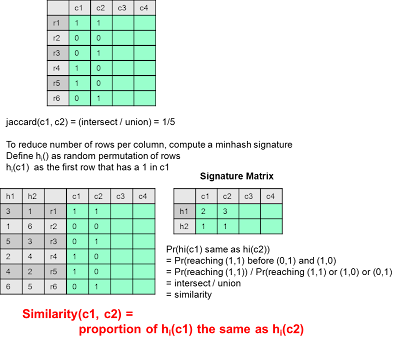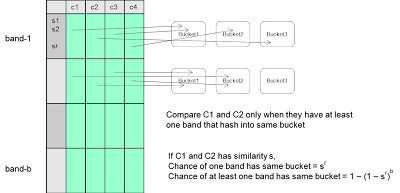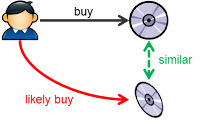## Thursday, September 1, 2011

### Recommendation Engine

In a classical model of recommendation system, there are "users" and "items". User has associated metadata (or content) such as age, gender, race and other demographic information. Items also has its metadata such as text description, price, weight ... etc. On top of that, there are interaction (or transaction) between user and items, such as userA download/purchase movieB, userX give a rating 5 to productY ... etc.Now given all the metadata of user and item, as well as their interaction over time, can we answer the following questions ...
1. What is the probability that userX purchase itemY ?
2. What rating will userX give to itemY ?
3. What is the top k unseen items that should be recommended to userX ?
Content-based Approach
In this approach, we make use of the metadata to categorize user and item and then match them at the category level. One example is to recommend jobs to candidates, we can do a IR/text search to match the user's resume with the job descriptions. Another example is to recommend an item that is "similar" to the one that the user has purchased. Similarity is measured according to the item's metadata and various distance function can be used. The goal is to find k nearest neighbors of the item we know the user likes.

Collaborative Filtering Approach
In this approach, we look purely at the interactions between user and item, and use that to perform our recommendation. The interaction data can be represented as a matrix.Notice that each cell represents the interaction between user and item. For example, the cell can contain the rating that user gives to the item (in the case the cell is a numeric value), or the cell can be just a binary value indicating whether the interaction between user and item has happened. (e.g. a "1" if userX has purchased itemY, and "0" otherwise.

The matrix is also extremely sparse, meaning that most of the cells are unfilled. We need to be careful about how we treat these unfilled cells, there are 2 common ways ...
• Treat these unknown cells as "0". Make them equivalent to user giving a rate "0". This may or may not be a good idea depends on your application scenarios.
• Guess what the missing value should be. For example, to guess what userX will rate itemA given we know his has rate on itemB, we can look at all users (or those who is in the same age group of userX) who has rate both itemA and itemB, then compute an average rating from them. Use the average rating of itemA and itemB to interpolate userX's rating on itemA given his rating on itemB.
User-based Collaboration Filter In this model, we do the following
1. Find a group of users that is “similar” to user X
2. Find all movies liked by this group that hasn’t been seen by user X
3. Rank these movies and recommend to user XThis introduces the concept of user-to-user similarity, which is basically the similarity between 2 row vectors of the user/item matrix. To compute the K nearest neighbor of a particular users. A naive implementation is to compute the "similarity" for all other users and pick the top K.

Different similarity functions can be used. Jaccard distance function is defined as the number of intersections of movies that both users has seen divided by the number of union of movies they both seen. Pearson similarity is first normalizing the user's rating and then compute the cosine distance.

There are two problems with this approach
1. Compare userX and userY is expensive as they have millions of attributes
2. Find top k similar users to userX require computing all pairs of userX and userY
Location Sensitive Hashing and Minhash
To resolve problem 1, we approximate the similarity using a cheap estimation function, called minhash. The idea is to find a hash function h() such that the probability of h(userX) = h(userY) is proportion to the similarity of userX and userY. And if we can find 100 of h() function, we can just count the number of such function where h(userX) = h(userY) to determine how similar userX is to userY. The idea is depicted as follows ...Notice the picking of h() depends on the picking of the distance function.  Our distance function above is based on Jaccard distance = 1 - (Intersect/Union), and our h() is minhash.  In case a difference distance function is used (e.g. cosine distance, euclidean distance), a different h() should be picked.

Also, computing a permutation of a large number of rows can be very expensive. Remember that the purpose of h(c1) is to return row number of the first row that is 1. So we can just scan each row of c1 to see if it is 1, if it is not, we can just ignore the row number as it can never be the minimum permuted row having 1.  But if the row of c1 is 1, then there is a chance that such a row being the minimum row under the permutation.  To simulate the permutation, we scan each row and apply a function newRowNum = hash(rowNum). Take the minimum of the newRowNum seen so far.

The algorithm is as followsNotice that hash() is different from h(), which is the minhash function.  Here we use hash() just to simulate the permutation.  For each h(), we pick two random number a, b.  And we define hash(x) as the universal hash function to be ((a.x + b)%p)%N where p is a prime number much bigger than N and N is 2^32.

To solve problem 2, we need to avoid computing all other users' similarity to userX. The idea is to hash users into buckets such that similar users will be fall into the same bucket. Therefore, instead of computing all users, we only compute the similarity of those users who is in the same bucket of userX.

The idea is to horizontally partition the column into b bands, each with r rows. By pick the parameter b and r, we can control the likelihood (function of similarity) that they will fall into the same bucket in at least one band.Item-based Collaboration Filter
If we transpose the user/item matrix and do the same thing, we can compute the item to item similarity. In this model, we do the following ...
1. Find the set of movies that user X likes (from interaction data)
2. Find a group of movies that is similar to these set of movies that we know user X likes
3. Rank these movies and recommend to user XIt turns out that computing item-based collaboration filter has more benefit than computing user to user similarity for the following reasons ...
• Number of items typically smaller than number of users
• While user's taste will change over time and hence the similarity matrix need to be updated more frequent, item to item similarity tends to be more stable and requires less update.
Singular Value Decomposition
The user to item matrix can be considered in dual form.  Each user is represented by a vector of items and each item is represented a vector of users.

Based on SVD, every matrix can be decomposed into 3 matrices.  Therefore we can decompose the user/item Matrix into 3 matrices.  This decomposition can be interpreted as there is a hidden (latent) space between users and items (called this the concept space).  The first one U can be considered as the User/Concept matrix, The diagonal matrix Σ can be considered as the scaling of concepts (according to the strength of each concept).  The V matrix can be considered as the Item/Concept matrix.

Therefore the user / item rating should be equivalent to the cosine similarity between a user vector and item vector in the concept space.

Notice that Σ can be thought as the strength of each "concept" in the concept space. And the value is order in terms of their magnitude in decreasing order. If we remove some of the weakest concept by making them zero, we reduce the number of non-zero elements in Σ, which effective generalize the concept space (make them focus in the important concepts).

Calculate SVD decomposition for matrix with large dimensions is expensive. Fortunately, if our goal is to compute an SVD approximation (with k diagonal non-zero value), we can use the random projection mechanism as describer here.

How to compute the user vector in the concept space ?
We can use a common space (ie: the item space) between user and concept, and then project the user along each concept vector.

One challenge of determining user similarity based on item space if that if the two users are viewing different set of movies without any overlapping, then they are not considered to be similar at all (even though the movies they view are similar in the concept space).  Therefore, instead of computing the cosine similarity of two user vectors in the item space, we can transform both user vector into the concept space and compute the cosine similarity there.

When a new user arrives, how do we predict his rating on all existing items ?
Basically, we just need to compute the cosine similarity between user vector and each item vector in the concept space.

Association Rule Based
In this model, we use the market/basket association rule algorithm to discover rule like ...
{item1, item2} => {item3, item4, item5}

We represent each user as a basket and each viewing as an item (notice that we ignore the rating and use a binary value). After that we use association rule mining algorithm to detect frequent item set and the association rules. Then for each user, we match the user's previous viewing items to the set of rules to determine what other movies should we recommend.

Evaluate the recommender
After we have a recommender, how do we evaluate the performance of it ?

The basic idea is to use separate the data into the training set and the test set. For the test set, we remove certain user-to-movies interaction (change certain cells from 1 to 0) and pretending the user hasn't seen the item. Then we use the training set to train a recommender and then fit the test set (with removed interaction) to the recommender. The performance is measured by how much overlap between the recommended items with the one that we have removed. In other words, a good recommender should be able to recover the set of items that we have removed from the test set.

Leverage tagging information on items
In some cases, items has explicit tags associated with them (we can considered the tags is a user-annotated concept space added to the items). Consider each item is described with a vector of tags. Now user can also be auto-tagged based on the items they have interacted. For example, if userX purchase itemY which is tagged with Z1, and Z2. Then user will increase her tag Z1 and Z2 in her existing tag vector. We can use a time decay mechanism to update the user's tag vector as follows ...

current_user_tag = alpha * item_tag + (1 - alpha) * prev_user_tag

To recommend an item to the user, we simply need to calculate the top k items by computing the dot product (ie: cosine distance) of the user tag vector and the item tag vector.